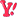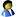Wrox Programmer ForumsHelp with efficient coding (part of Dynamic Query
 |
 SQL Language SQL Language discussions not specific to a particular RDBMS program or vendor.
 Welcome to the p2p.wrox.com Forums. You are currently viewing the SQL Language section of the Wrox Programmer to Programmer discussions. This is a community of software programmers and website developers including Wrox book authors and readers. New member registration was closed in 2019. New posts were shut off and the site was archived into this static format as of October 1, 2020. If you require technical support for a Wrox book please contact http://hub.wiley.com

 miamikkAuthorized User Join Date: Mar 2005 Posts: 14 Thanks: 0 Thanked 0 Times in 0 PostsHelp with efficient coding (part of Dynamic Query

I need help with this code in making it more efficient (part of Dynamic SQL query inside Stored Procedure). The code takes about 2-3 min to generate output. I am a newbie to SQL programming and I would appreciate if anyone can rewirite the code for the solution I need.

The output of this code needs to generate a table with columns from present year data plus columns with previous 4 years of data. Each column values are calculated from that particular year table & its prevoius year table. The data tables are named sequentially as (2000exp, 2001exp ....2005exp, 2006exp). The final output should like the image below.

Image1

I wrote the code that calculates values for 1 column and I am just repeating the same code 4 times for remaining 4 other columns. This definitely not an efficient way as most of the code is repeated. The code is shown in the image below. I highlighted repeatitive code with same colors

Image2

The main stored procedure will get 3 inputs (tablename, month and district like 2006exp, 12, 1). Part of complete SP code is shown in image below. The part of code I need help will be for case 0.

Image3

I would appreciate if anyone can help me. The code that generates values for 1 column is below.

Select ty.Amount1 as Amount, ((ty.Amount1-py.Amount2)/py.Amount2)*100 as GrowthRate,
(ty.Amount1/ty.Total1)*100 as Share, ty.Total1 as Total, ((ty.Total1-py.Total2)/py.Total2)*100 as Total_GrowthRate
from
(Select a.district, Sum(a.all_val_mo) as Amount1, (select Sum(a1.all_val_mo) FROM [2006exp] a1 where a1.stat_month <=1) as Total1
FROM [2006exp] a where a.stat_month=1 and a.district=1
Group by a.district) ty
JOIN
(Select b.district, Sum(b.all_val_mo) as Amount2, (select Sum(b1.all_val_mo) FROM [2005exp] b1 where b1.stat_month <=1) as Total2
FROM [2005exp] b where b.stat_month=1 and b.district=1
Group by b.district) py on ty.district=py.district

Image4

 bdyc2007Registered User Join Date: Jul 2007 Posts: 2 Thanks: 0 Thanked 0 Times in 0 PostsJust for Image2, the code can be updated like below:

declare @a int
declare @b int
declare @c int
declare @d int
declare @e int
declare @f int
declare @g int
declare @h int

select @a=sum(all_val_no) from [2006exp]
select @b=sum(all_val_no) from [2005exp]
select @e=sum(all_val_no) from [2004exp]
select @g=sum(all_val_no) from [2003exp]

select @c=sum(all_val_no) from [2006exp] where stat_month<=12
select @d=sum(all_val_no) from [2005exp] where stat_month<=12
select @f=sum(all_val_no) from [2004exp] where stat_month<=12
select @h=sum(all_val_no) from [2003exp] where stat_month<=12

insert into TestTable
select @a as Amount,((@a-@b)/@b)*100 as GrowthRate,(@a/@c)*100 as Share,@c as Total, ((@c-@d)/@d)*100 as Total_GrowthRate

insert into TestTable
select @a as Amount,((@b-@e)/@b)*100 as GrowthRate,(@b/@d)*100 as Share,@d as Total, ((@d-@f)/@f)*100 as Total_GrowthRate

insert into TestTable
select @a as Amount,((@e-@g)/@b)*100 as GrowthRate,(@e/@f)*100 as Share,@f as Total, ((@f-@h)/@h)*100 as Total_GrowthRate

...

And you'd better change a,b,c...to the fittable name of parameter.

The code means, after you calculating 2006 and 2005, when you need to calculate 2005 and 2004, you just need to calculate 2004 ,no 2005.

I think use this method can save some time.

And in my opinion, it's not good for the system performance when using so many 'JOIN'.Similar Threads Thread Thread Starter Forum Replies Last Post Nested Query with an iif statment part 2 Corey Access 1 October 17th, 2005 12:47 PM Coding help with data from a query Gus Access VBA 3 April 15th, 2005 06:32 AM Dynamic control part 2 Warbird General .NET 0 July 12th, 2004 08:50 AM how to make the following query as efficient as po hollyhyl SQL Language 1 May 28th, 2004 10:26 PM A query to extract part of a string RayL Access 3 March 10th, 2004 09:42 AM